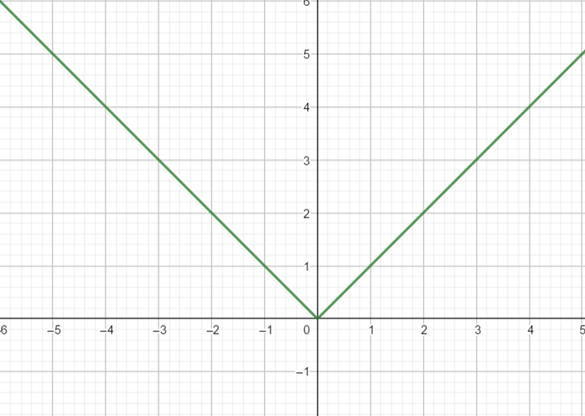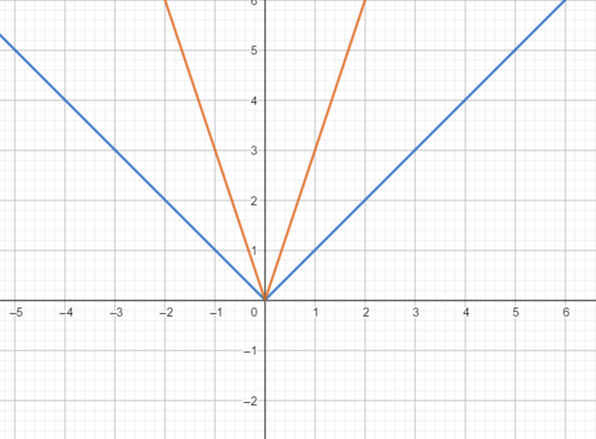# To graph: the function f ( x ) = 3 | x | using the graph of a standard function and then applying transformations.### Precalculus: Mathematics for Calcu...

6th Edition
Stewart + 5 others
Publisher: Cengage Learning
ISBN: 9780840068071### Precalculus: Mathematics for Calcu...

6th Edition
Stewart + 5 others
Publisher: Cengage Learning
ISBN: 9780840068071

#### Solutions

Chapter 2.5, Problem 35E
To determine

## To graph: the function f(x)=3|x| using the graph of a standard function and then applying transformations.

Expert Solution

### Explanation of Solution

Given information:

the function is f(x)=3|x|

Graph:

To plot the graph of f(x)=|x| ,use the plotting points method, substitute different value of x on f(x)=|x| to determine corresponding value of f(x) .

The table is thus obtained as:

 x -2 -1 1 2 y 2 1 1 2

now plot each point of x along the horizontal axis and the corresponding value of f(x)=y along vertical axis and connect each point to form a smooth curve.

The graph of f(x)=|x| is obtained as:Now, the graph of f(x)=3|x| is the graph of f(x)=|x| stretchedvertically by a factor of 3 .

The graphof f(x)=3|x| is obtained as:Interpretation:

From the above graph it can be obtained that the graph of f(x)=3|x| is obtained by stretching the graph of f(x)=|x| vertically by a factor of 3 .

### Have a homework question?

Subscribe to bartleby learn! Ask subject matter experts 30 homework questions each month. Plus, you’ll have access to millions of step-by-step textbook answers!